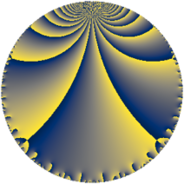# Properties

 Label 45.4.fLevel $45$ Weight $4$ Character orbit 45.f Rep. character $\chi_{45}(8,\cdot)$ Character field $\Q(\zeta_{4})$ Dimension $12$ Newform subspaces $1$ Sturm bound $24$ Trace bound $0$

# Related objects

## Defining parameters

 Level: $$N$$ $$=$$ $$45 = 3^{2} \cdot 5$$ Weight: $$k$$ $$=$$ $$4$$ Character orbit: $$[\chi]$$ $$=$$ 45.f (of order $$4$$ and degree $$2$$) Character conductor: $$\operatorname{cond}(\chi)$$ $$=$$ $$15$$ Character field: $$\Q(i)$$ Newform subspaces: $$1$$ Sturm bound: $$24$$ Trace bound: $$0$$

## Dimensions

The following table gives the dimensions of various subspaces of $$M_{4}(45, [\chi])$$.

Total New Old
Modular forms 44 12 32
Cusp forms 28 12 16
Eisenstein series 16 0 16

## Trace form

 $$12 q + 24 q^{7} + O(q^{10})$$ $$12 q + 24 q^{7} + 192 q^{10} - 108 q^{13} - 648 q^{16} + 1056 q^{22} - 144 q^{25} - 576 q^{28} - 1248 q^{31} + 828 q^{37} + 2568 q^{40} - 96 q^{43} + 672 q^{46} - 312 q^{52} - 1512 q^{55} - 3864 q^{58} + 96 q^{61} + 1632 q^{67} - 1536 q^{70} + 3972 q^{73} - 480 q^{76} - 7848 q^{82} - 1752 q^{85} + 7968 q^{88} + 4752 q^{91} + 2772 q^{97} + O(q^{100})$$

## Decomposition of $$S_{4}^{\mathrm{new}}(45, [\chi])$$ into newform subspaces

Label Dim $A$ Field CM Traces $q$-expansion
$a_{2}$ $a_{3}$ $a_{5}$ $a_{7}$
45.4.f.a $12$ $2.655$ $$\mathbb{Q}[x]/(x^{12} - \cdots)$$ None $$0$$ $$0$$ $$0$$ $$24$$ $$q+\beta _{6}q^{2}+(-6\beta _{1}+\beta _{5})q^{4}+(\beta _{9}+\beta _{10}+\cdots)q^{5}+\cdots$$

## Decomposition of $$S_{4}^{\mathrm{old}}(45, [\chi])$$ into lower level spaces

$$S_{4}^{\mathrm{old}}(45, [\chi]) \cong$$ $$S_{4}^{\mathrm{new}}(15, [\chi])$$$$^{\oplus 2}$$